Back to Aurora Vision Library website

You are here: Start » Function Reference » Data Classification

# Data Classification

## Clustering

Icon Name Description / Applications Modules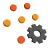ClusterData_KMeans

Clusters data using KMeans algorithm.

FoundationProClusterPoints2D

Clusters 2D points using K Means Clustering method.

FoundationProClusterPoints2D_SingleLink

Clusters data using hierarchical single-link algorithm.

FoundationProClusterPoints3D

Clusters 3D points using K Means Clustering method.

FoundationProFindConnectedComponents

Finds connected components in a graph given as set of bidirectional connections.

FoundationPro

## Data Classification Common

Icon Name Description / Applications Modules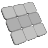CreateDataPartition

Divides the input set to test and train subsets, trying to maintain balance in class distribution.

FoundationProMeasureClassificationQuality_Binary

Calculates classification performance metrics for binary problems.

FoundationProMeasureClassificationQuality_Multiclass

Calculates classification performance metrics for multiclass problems.

FoundationPro

## Multilayer Perceptron

Icon Name Description / Applications Modules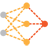MLP_Init

Creates multilayer perceptron model.

FoundationProMLP_Respond

Calculates multilayer perceptron answer.

FoundationPro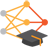MLP_Train

Creates and trains multilayer perceptron classifier.

FoundationPro

## Nearest Neighbors

Icon Name Description / Applications Modules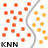KNN_Classify

Classify data using the KNN classifier.

FoundationPro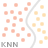KNN_Init

Initializes the KNN classifier.

FoundationPro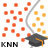KNN_Train

Trains KNN classifier using sample data.

FoundationPro

## Principal Component Analysis

Icon Name Description / Applications Modules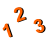ApplyPCATransform

Applies previously obtained Principal Component Analysis (PCA) transformation coefficients to new data.

FoundationProCreatePCATransform

Performs the Principal Component Analysis (PCA) on provided data, creates the feature vector and normalization coefficients (mean and standard deviation of variables).

FoundationProMatrixDeterminant

Find the determinant of a square matrix.

FoundationProMatrixPseudoEigenvectors

Find the pseudo-eigenvalues and pseudo-eigenvectors of a symmetrical square matrix.

FoundationProNormalizeMatrixData

Treats Matrix as a data frame, where examples are in rows while columns represent features, and normalizes the data by subtracting mean from each column and dividing it by its standard deviation.

FoundationProReversePCATransform

Reverses Principal Component Analysis (PCA) process. Can be used to transform data back to original feature space.

FoundationPro

## Regression Analysis

Icon Name Description / Applications ModulesLinearRegression

Computes linear regression of given point set.

FoundationBasicLinearRegression_LTE

Computes linear regression of given point set using Least Trimmed Error algorithm.

FoundationProLinearRegression_M

Computes linear regression of given point set using selected M-estimator for outlier suppression.

FoundationProLinearRegression_RANSAC

Computes linear regression of given point set using RANSAC.

FoundationProLinearRegression_TheilSen

Computes linear regression of given point set using TheilSen algorithm.

FoundationBasicQuadraticRegression

Computes quadratic regression of given point set.

FoundationBasicQuadraticRegression_M

Computes quadratic regression of given point set using selected M-estimator for outlier suppression.

FoundationProQuadraticRegression_RANSAC

Computes quadratic regression of given point set using RANSAC.

FoundationProStatistics_OfArray

Computes basic statistical information out of an array of real numbers. The array must be not empty.

FoundationBasicStatistics_OfLoop

Computes basic statistical information out of real numbers appearing in consecutive iterations.

FoundationBasic

## Statistics

Icon Name Description / Applications ModulesFindDataMode_FixedCount

Finds the mode in a set of data values by looking for highest concentration of a fixed number of samples.

Can be used to determine a histogram maximum without actually creating the histogram.

FoundationProFindDataMode_FixedSpread

Finds the mode in a set of data values by looking for highest number of samples withing the specified spread.

Can be used to determine a histogram maximum without actually creating the histogram.

FoundationProFindDataMode_MeanShift

Finds the mode in a set of data values by iteratively computing its median.

Can be used to determine a histogram maximum without actually creating the histogram.

FoundationProFindDensityMaxima_FixedCount

Finds local density maxima in set of values by looking for the highest concentration of a fixed number of samples.

Can be used to determine histogram's local maxima without actually creating the histogram.

FoundationProFindDensityMaxima_FixedSpread

Finds local density maxima in a set of values by looking for the highest number of samples withing a range determined by the given spread.

Can be used to determine histogram's local maxima without actually creating the histogram.

FoundationProFindMatchingRegions_IoU

Finds corresponding regions in two arrays based on IoU value.

FoundationProRegionsIoU

Computes intersection over union value for two regions.

FoundationProTableOfConfusion_Basic

Computes statistics from a confusion matrix for given TP, FP, TN, FN.

FoundationProTableOfConfusion_BoolArray

Computes statistics from a confusion matrix for an array of groundTruth and results.

FoundationProTableOfConfusion_Histograms

Computes confusion matrix based on two histograms and threshold value.

FoundationProTableOfConfusion_Images

Computes statistics from a confusion matrix for image of groundTruth and results.

FoundationPro

## Support Vector Machines

Icon Name Description / Applications Modules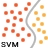SVM_ClassifyMultiple

Classifies input points based on trained model.

FoundationProSVM_ClassifySingle

Classifies input features based on a trained model.

FoundationPro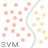SVM_Init

Initializes an SVM model.

FoundationPro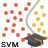SVM_Train

Trains an SVM model.

FoundationPro